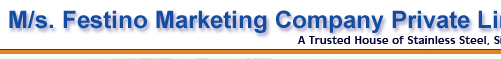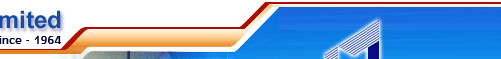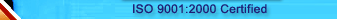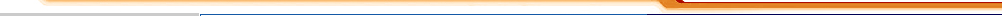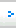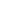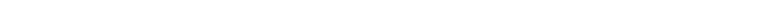..:: Calculation Formula ::.. Weight of S.S. Pipe OD (MM) - W.T. (MM) X W.T. (MM) X 0.00756 = Kg (Per Ft) OD (MM) - W.T. (MM) X W.T. (MM) X 0.0248 = Kg (Per Mtr)   Weight of S.S. Round Dia (MM) X Dia (MM) X 0.0019 Kg (Per Ft)   Weight of S.S. Hexazonal Road Dia (MM) X Dia (MM) X 0.002072 Kg (Per Ft)   Weight of S.S. Square Rod Diameter X Diameter X 0.0025 = Weight Per Ft   Weight of S.S. Sheets (A) Length (mtr) X Width (mtr) X Thickness (mtr) X 8 = Kg (Per Sheet)   Weight of S.S. Circle in MM per Piece Diameter X Diameter X Thickness / 160000 = Weight Per Pc   Weight of S.S. Flat in MM Per Ft Width X -304 X Thickness X 8 = Weight Per Ft   Weight of Copper Pipe OD (MM) - W.T. (MM) X W.T. (MM) X 0.0078 = Kg Per Ft   Weight of S.S. Sheets, Plate, Pipe, Round, Hex, Circle, Square, Flat / 3 = Aluminium Weight Approx   Making of Pipe from Sheet or Plate OD - Thick X 3.14 = Width of Cutting Sheet of Plate   Pressure Conversion Kg/mm2 X 1422.3 = Psi = Kg / cm2 X 14.223   1 Feet = 0.3048 Meter 1 Meter = 3.2808 Feet 1 Inch = 25.4 MM 1 Pound = 0.454 (45359243) Kgs

 All Rights Reserved. Festinosteel.com Best View at 1024 x 768 pixels